# Copy a Formula

When you copy a formula, Excel automatically adjusts the cell references for each new cell the formula is copied to.

### Copy 101

Simply use CTRL + c and CTRL + v to copy and paste a formula in Excel.

1. For example, to copy a formula, select cell A3 below and press CTRL + c.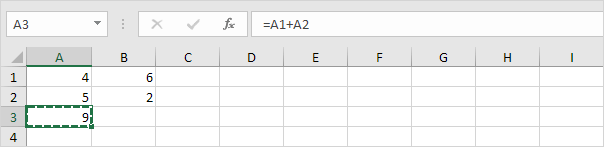2. To paste this formula, select cell B3 and press CTRL + v.3. Click in the formula bar to clearly see that the formula references the values in column B.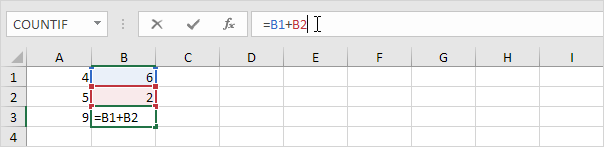### Fill Handle

Use the fill handle in Excel to quickly copy a formula to other cells.

1. For example, select cell A3 below, click on the lower right corner of cell A3 (the fill handle) and drag it across to cell F3.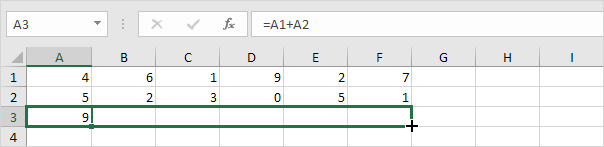Result.You can also use the fill handle to quickly copy a formula down a column.

2. For example, select cell C1 below, click on the lower right corner of cell C1 (the fill handle) and drag it down to cell C6.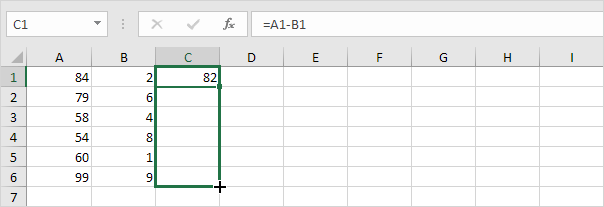Result.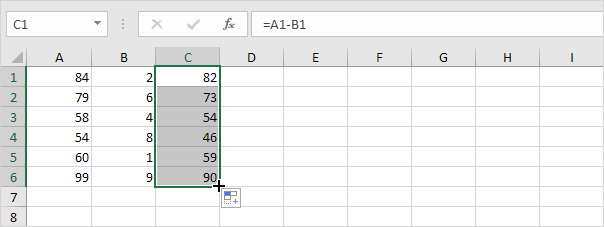Tip: instead of dragging the fill handle down, simply select cell C1 and double click the fill handle. If you have hundreds of rows of data, this can save time!

### Absolute Reference

Create an absolute reference to fix the reference to a cell or range of cells. When you copy a formula, an absolute reference never changes.

1. For example, fix the reference to cell E2 below by placing a \$ symbol in front of the column letter and row number.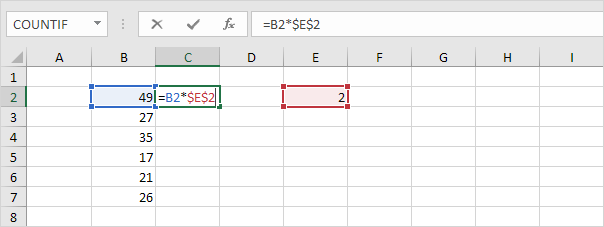2. Select cell C2, click on the lower right corner of cell C2 and drag it down to cell C7.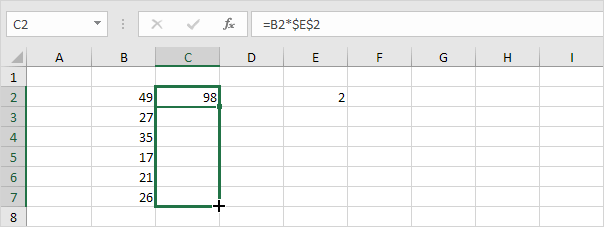Check: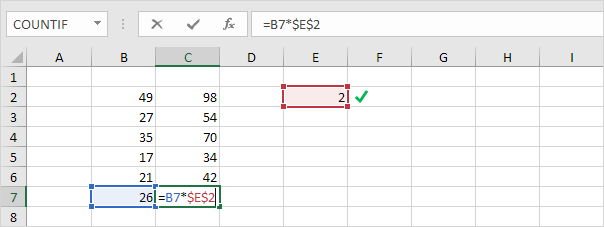### Move a Formula

To move a formula in Excel, simply use cut (CTRL + x) and paste (CTRL + v). Excel pros use the following trick to move a formula.

1. Select a cell with a formula.

2. Hover over the border of the selection. A four-sided arrow appears.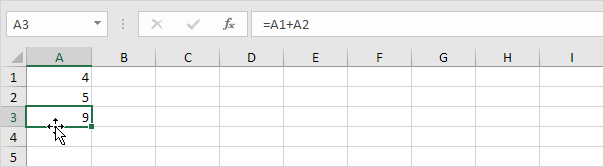3. Click and hold the left mouse button.

4. Move the formula to the new position.5. Release the left mouse button.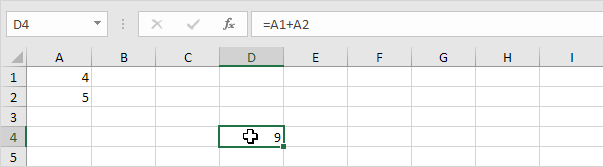### Exact Copy

To make an exact copy of a formula, without changing the cell references, execute the following easy steps.

1. Click in the formula bar and select the formula.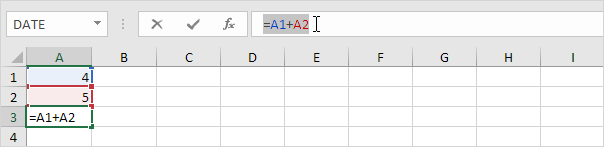2. Press CTRL + c and press Enter.

3. Select another cell and press CTRL + v.

Result: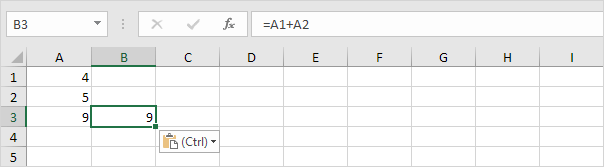Conclusion: cell A3 and cell B3 contain the exact same formula.

### Copy Magic

To make an exact copy of multiple formulas, repeat the previous steps for each formula. You can also use the following magic trick.

1. Select multiple formulas.2. Replace all equal signs with xxx.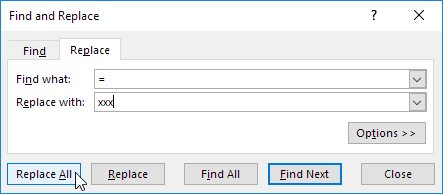Result.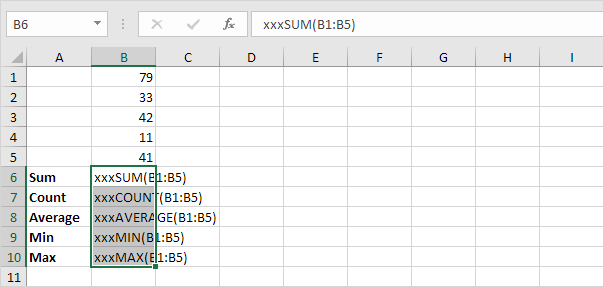3. Use CTRL + c and CTRL + v to copy and paste the text strings.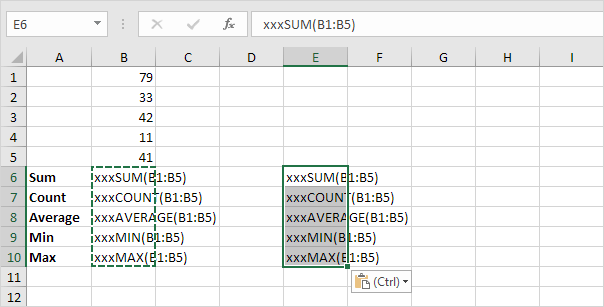4. Select the range B6:B10, hold down CTRL, select the range E6:E10 and replace all occurrences of 'xxx' with equal signs (the exact opposite of step 2).

Result.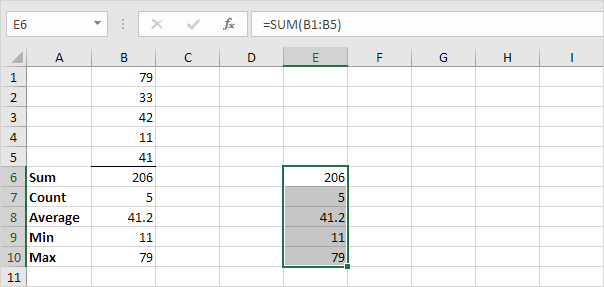Conclusion: cell B6 and cell E6 contain the exact same formula, cell B7 and cell E7 contain the exact same formula, etc.

Go to Next Chapter: Date & Time Functions